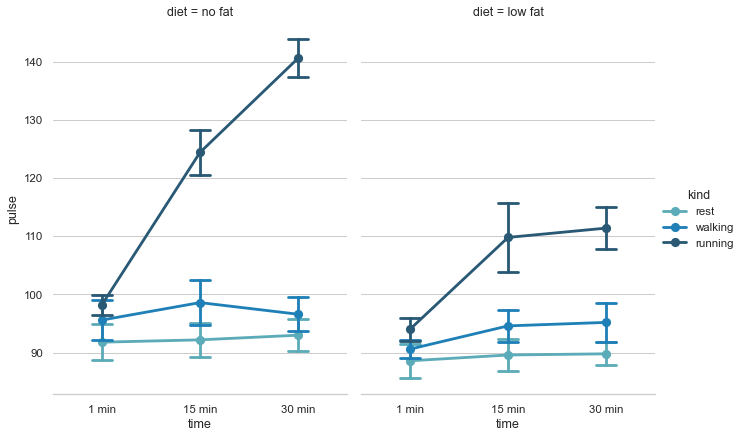# Plotting a three-way ANOVA¶seaborn components used: `set_theme()`, `load_dataset()`, `catplot()`

```import seaborn as sns
sns.set_theme(style="whitegrid")

# Load the example exercise dataset
df = sns.load_dataset("exercise")

# Draw a pointplot to show pulse as a function of three categorical factors
g = sns.catplot(x="time", y="pulse", hue="kind", col="diet",
capsize=.2, palette="YlGnBu_d", height=6, aspect=.75,
kind="point", data=df)
g.despine(left=True)
```